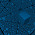Friday, February 17, 2012

IIT-JEE Multiple Correct Answer Type Questions on Thermodynamics

"Hate the sin, love the sinner."
–Mahatma Gandhi

Today we will discuss two multiple correct answer type questions on thermodynamics. These were included in the IIT-JEE 2009 question paper:(1) The figure shows the P–V plot of an ideal gas taken through a cycle ABCDA. The part ABC is a semi-circle and CDA is half of an ellipse. Then,

(A) the process during the path A B is isothermal

(B) heat flows out of the gas during the path B C D

(C) work done during the path A B C is zero

(D) positive work is done by the gas in the cycle ABCDA.

Statement (A) is incorrect since a curve representing an isothermal in a PV diagram must be a hyperbola and not an arc of a circle.

We have PV = nRT with usual notations. The value of V is smaller at D compared to that at B where as the value of P is the same. The product PV = nRT is therefore smaller at D. This means that the temperature at D is less than that at B and hence statement (B) is correct.

During the path AB the gas expands, doing work. During the path BC the gas is compressed and work is done on the gas. But these works are unequal since the area under AB is greater than the area under BC. Therefore, statement (c) is incorrect.

The cycle of operations ABCDA is clockwise and the cyclic curve encloses an area. Therefore, positive work is done by the gas and statement (D) is correct.

So the correct options are (B) and (D).

(2) CV and CP denote the molar specific heat capacities of a gas at constant volume and constant pressure respectively. Then

(A) CP – CV is larger for a diatomic ideal gas than for a mono-atomic ideal gas

(B) CP + CV is larger for a diatomic ideal gas than for a mono-atomic ideal gas

(C) CP/CV is larger for a diatomic ideal gas than for a mono-atomic ideal gas

(D) CP.CV is larger for a diatomic ideal gas than for a mono-atomic ideal gas.

In the case of ideal gases CP – CV = R, the universal gas constant. Therefore statement (A) is wrong.

CP and CV are larger for a diatomic ideal gas compared to a mono-atonic ideal gas. Therefore, CP + CV is larger for a diatomic ideal gas. Statement (B) is correct.

[For diatomic ideal gas CP = 7R/2 and CV = 5R/2. For mono-atomic ideal gas CP = 5R/2 and CV = 3R/2].

Obviously statement (C) is wrong.

Statement (D) is correct since both CP and CV are larger for a diatomic ideal gas than for a mono-atomic ideal gas.

Therefore, options (B) and (D) are correct.

You will find a useful post on thermodynamics here.

1.2.